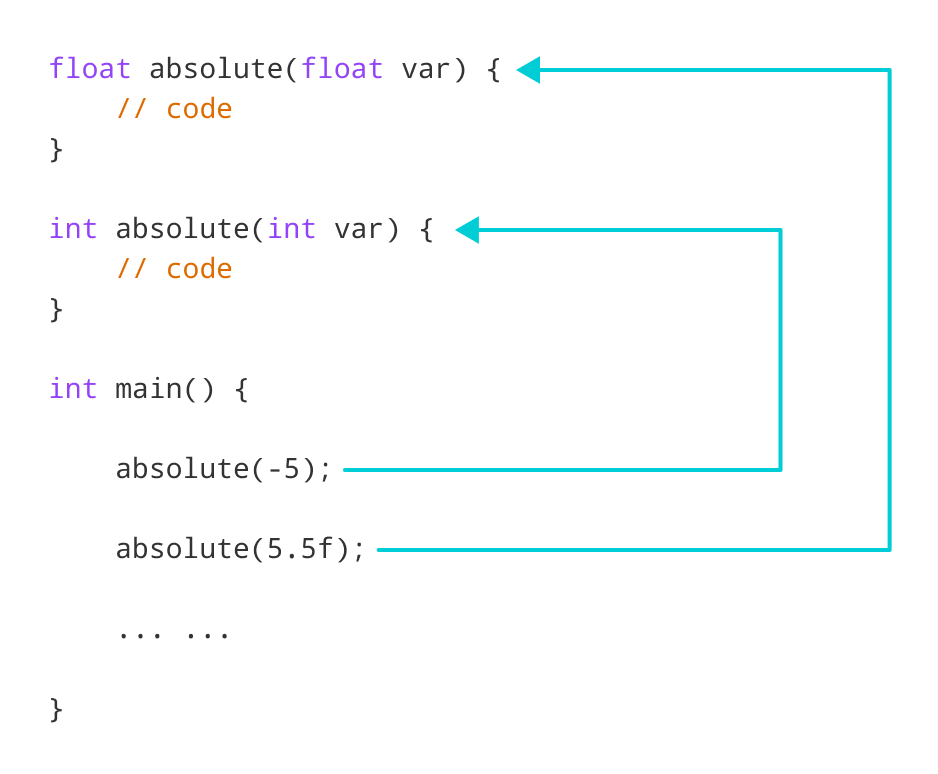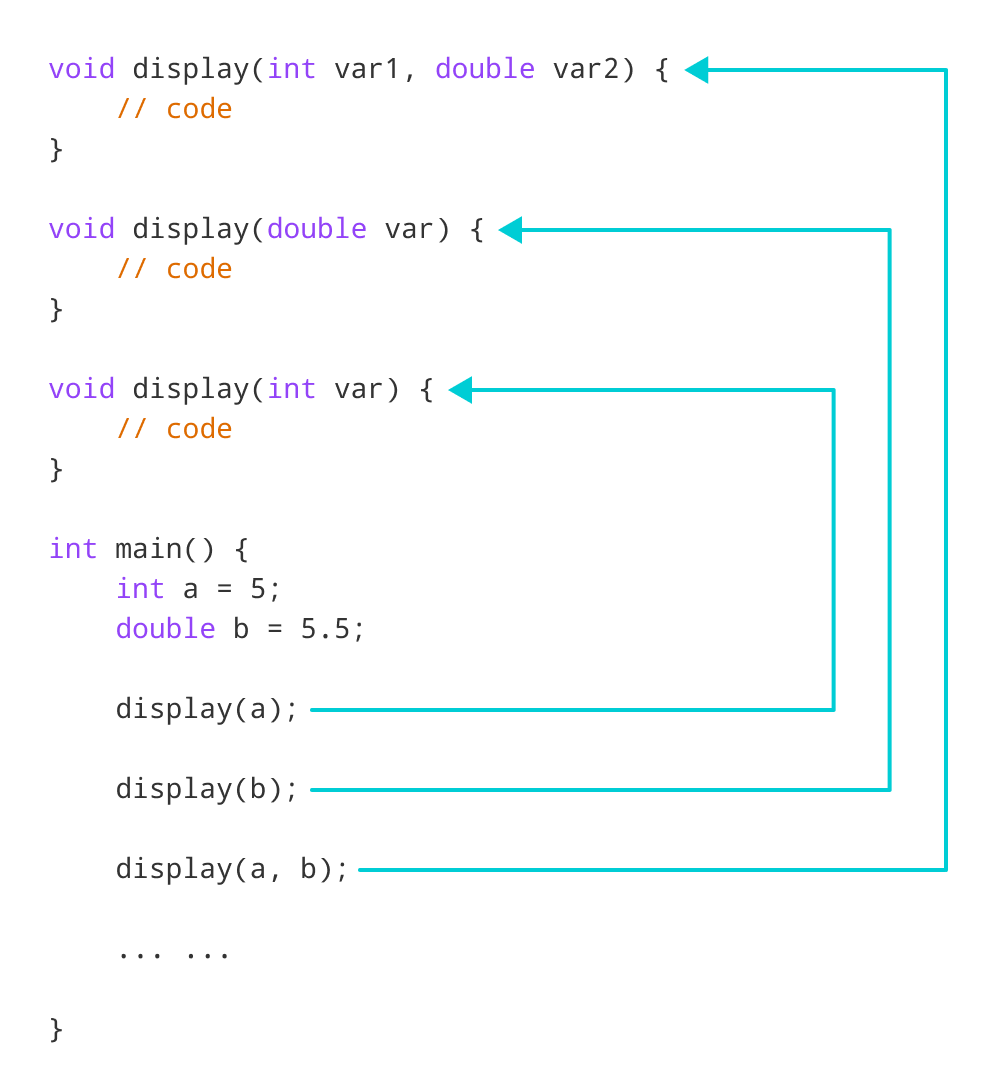# C++ Function Overloading

In this tutorial, we will learn about the function overloading in C++ with examples.

In C++, two functions can have the same name if the number and/or type of arguments passed is different.

These functions having the same name but different arguments are known as overloaded functions. For example:

``````// same name different arguments
int test() { }
int test(int a) { }
float test(double a) { }
int test(int a, double b) { }``````

Here, all 4 functions are overloaded functions.

Notice that the return types of all these 4 functions are not the same. Overloaded functions may or may not have different return types but they must have different arguments. For example,

``````// Error code
int test(int a) { }
double test(int b){ }``````

Here, both functions have the same name, the same type, and the same number of arguments. Hence, the compiler will throw an error.

## Example 1: Overloading Using Different Types of Parameter

``````// Program to compute absolute value
// Works for both int and float

#include <iostream>
using namespace std;

// function with float type parameter
float absolute(float var){
if (var < 0.0)
var = -var;
return var;
}

// function with int type parameter
int absolute(int var) {
if (var < 0)
var = -var;
return var;
}

int main() {

// call function with int type parameter
cout << "Absolute value of -5 = " << absolute(-5) << endl;

// call function with float type parameter
cout << "Absolute value of 5.5 = " << absolute(5.5f) << endl;
return 0;
}``````

Output

```Absolute value of -5 = 5
Absolute value of 5.5 = 5.5```Working of overloading for the absolute() function

In this program, we overload the `absolute()` function. Based on the type of parameter passed during the function call, the corresponding function is called.

## Example 2: Overloading Using Different Number of Parameters

``````#include <iostream>
using namespace std;

// function with 2 parameters
void display(int var1, double var2) {
cout << "Integer number: " << var1;
cout << " and double number: " << var2 << endl;
}

// function with double type single parameter
void display(double var) {
cout << "Double number: " << var << endl;
}

// function with int type single parameter
void display(int var) {
cout << "Integer number: " << var << endl;
}

int main() {

int a = 5;
double b = 5.5;

// call function with int type parameter
display(a);

// call function with double type parameter
display(b);

// call function with 2 parameters
display(a, b);

return 0;
}``````

Output

```Integer number: 5
Float number: 5.5
Integer number: 5 and double number: 5.5```

Here, the `display()` function is called three times with different arguments. Depending on the number and type of arguments passed, the corresponding `display()` function is called.Working of overloading for the display() function

The return type of all these functions is the same but that need not be the case for function overloading.

Note: In C++, many standard library functions are overloaded. For example, the `sqrt()` function can take `double`, `float`, `int,` etc. as parameters. This is possible because the `sqrt()` function is overloaded in C++.